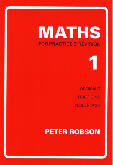# Maths for Practice & RevisionA set of five books covering basic mathematical techniques up to Common Entrance, public school scholarship and GCSE. Each double-page spread features a separate topic. The left hand page explains the method(s) involved, together with worked examples, and the right hand page gives related questions for the student to try. Thus the student requires little or no help from the teacher, making the series particularly useful for revision at home. At the end of each book there are fifty mixed questions of the types found on earlier pages. There is also a separate book which gives the answers to all the questions in the series.

Product Image Item Name- Price### MATHS for Practice and Revision 1

Decimal columns; four rules of decimals; converting decimals to fractions and vice versa; arranging decimals and fractions in order of size;...
£3.95### MATHS for Practice and Revision 2

Prime factors; HCF and LCM; squares and square roots; unitary sums; ratio; linear measurement; area and volume; estimation and approximation;...
£3.95### MATHS for Practice and Revision 3

(Algebra) Directed numbers; four rules of algebra; brackets; simple factorisation; substitution; equations and inequalities; formulae. Suitable for...
£3.95### MATHS for Practice and Revision 4

24-hour clock; time problems; set notation and Venn diagrams; distance, speed and time; bases other than 10; angles, parallel lines; polygons....
£3.95### MATHS for Practice and Revision 5

Pythagoras' theorem; simultaneous equations; matrices; vectors; multiplication of two brackets; quadratic equations; probability; average (mean,...
£3.95